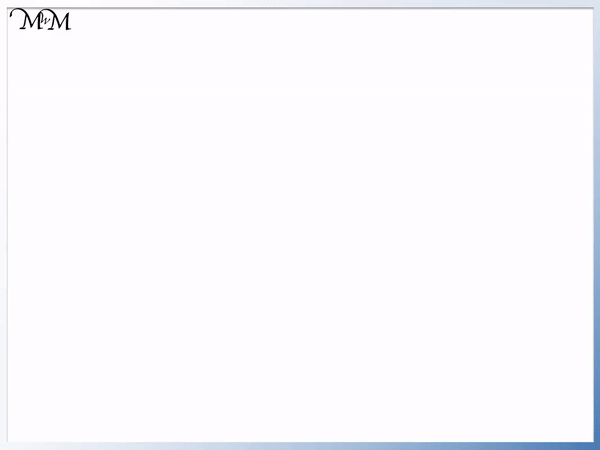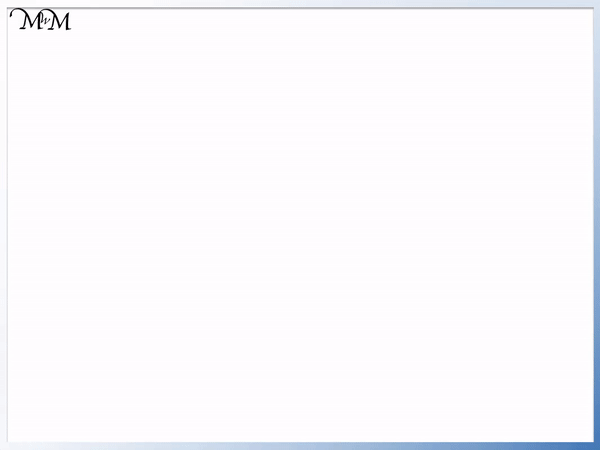# Comparing 2-Digit Numbers with Greater Than and Less Than

Comparing 2-Digit Numbers with Greater Than and Less Than• Here are two numbers: 42 and 49.
• To decide which number is larger, look at the digits in each place value column from left to right.
• The order of digits from smallest to largest is: 0, 1, 2, 3, 4, 5, 6, 7, 8 and 9.
• Both 42 and 49 have a ‘4’ in the tens column as their first digit.
• Because this digit is the same, we compare the next digit.
• ‘2’ is smaller than ‘9’ in the order of digits.
• Therefore 42 is less than 49.
• We can write this using the ‘less than’ symbol: ‘<'.
• The less than symbol ‘<' points at the smaller number of 42.

Compare the digits in each place value column from left to right.

We write a comparison symbol: ‘<' or '>‘ between the two numbers so that the symbol points to the smaller number.• We are comparing the numbers 61 and 29.
• The order of digits from smallest to largest is: 0, 1, 2, 3, 4, 5, 6, 7, 8 and 9.
• 6 is a larger digit than 2.
• Therefore 61 is greater than 29.
• The comparison symbol for ‘greater than’ is ‘>’, pointing to the smaller number of 29.Supporting Lessons# Comparing 2-Digit Numbers using Less Than and Greater Than Symbols

Each number is made up of digits.

The order of digits from least to greatest is:

0, 1, 2, 3, 4, 5, 6, 7, 8 and 9

A two-digit whole number is a number that is made up of two digits next to each other, with one digit in the tens column and one digit in the units column.

The smallest two-digit whole number is 10 and the largest is 99.

In this lesson we will learn how to compare the sizes of all numbers up to 100.

To compare the size of whole numbers use the following steps:

• A whole number is larger than another if it has more digits.
• If the number of digits in each number is the same, then look at the digits from left to right.
• If the left digit is larger in one number, then this is the largest number.
• If this digit is the same, compare the next digit along on the right.

In the example below we have 15 and 4.

We can count the number of digits in each number.15 is a two-digit number because it is made of two digits: ‘1’ and ‘5’.

4 is a one-digit number because it is only made of one digit: ‘4’.

If a whole number has more digits than another whole number then it is larger.

15 is greater than 4.

This is because one-digit numbers are all smaller than 10 and all two digit numbers must contain at least one lot of 10.

We can see that 15 is greater than 4 just by counting the digits in this number.

This method will only work for whole numbers and will not work for decimal numbers.

We can use comparison symbols to write ‘greater than’ or ‘less than’ more easily and quickly.

• ‘<' is the comparion symbol which is read as 'less than'
• ‘>’ is the comparison symbol which is read as ‘greater than’

15 is greater than 4

in words, it can be easier to just write:

15 > 4

To help remember which way around to write the comparison symbol, we can remember that the open end of ‘>’ or ‘<' is like an open crocodile's mouth.

The crocodile wants to eat the larger number.

An alternative way is to remember that the ‘pointy end’ of each symbol is like an arrowhead and we always point to the smaller number.

15 > 4

In the example below we have 18 and 38.

Both 18 and 38 have two digits and so we need to look at each place value column from left to right.The first digit in each number is in the tens column.

‘1’ is a smaller digit than ‘3’

And so,

18 is less than 38

We can write this by replacing ‘less than’ with the comparison symbol for less than, which is: ‘<'.

18 < 38

Remember that the symbol points to the smaller number.

In this example 18 has ‘1’ in the tens column, which means that it is larger than 10 but less than 20.

38 has a ‘3’ in the tens column, which means that is is larger than 30 but less than 40.

In the example below we are comparing 61 and 29.

Both 61 and 29 are two-digit numbers.We compare these numbers by comparing the size of each digit from left to right.

‘6’ is larger than ‘2’ and so 61 is greater than 29.

We write this as 61 > 29.

‘>’ is read as ‘greater than’ and we can remember this by thinking of the symbol as an arrow pointing at the smaller number of 29.

We were able to compare the two numbers by looking at the first digit.

We do not look at the second digit at all.

We know that 61 is larger than 60 because it has 6 tens.

We know that 29 is less than 30 because it does not have three tens. It only has 2 tens in the tens column.

In the next example of comparing two-digit numbers, we have 77 and 92.‘7’ is less than ‘9’ and so 77 is less than 92.

We can write 77 < 92.

In the final example we are comparing 42 and 49.

Both numbers have 2 digits and so we begin by comparing the digits from left to right.Both numbers have a ‘4’ in the tens digit.

Because the digit is the same we need to look at the next digit in both numbers to compare them.

42 has a ‘2’ in the units column.

49 has a ‘9’ in the units column.

2 is smaller than 9 in the order of digits.

And so, 42 is less than 49.

We write 42 < 49.

The comparison symbol points to the smaller number.

Both numbers are in the forties.

Both numbers have a 4 in the tens column which means that they are both greater than 40 but less than 50.

We had to look at the units column to decide which number was larger.Now try our lesson on Reading Numbers in the Thousands where we learn how to read three-digit numbers that are greater than one-thousand.